# Jarrow-Rudd Model in Excel

This is part 5 of the Binomial Option Pricing Excel Tutorial.

In the previous parts we have created binomial trees in our spreadsheet and implemented Cox-Ross-Rubinstein Excel formulas for up and down move sizes and probabilities.

In this part we will adjust the formulas for another binomial model, Jarrow-Rudd.

## Extending the Spreadsheet for Multiple Models

As we have already explained, most of the calculations (including the entire underlying price and option price tree) are the same for different binomial models. The only difference is the up and down move sizes and probabilities, which we calculate in cells B15-B18.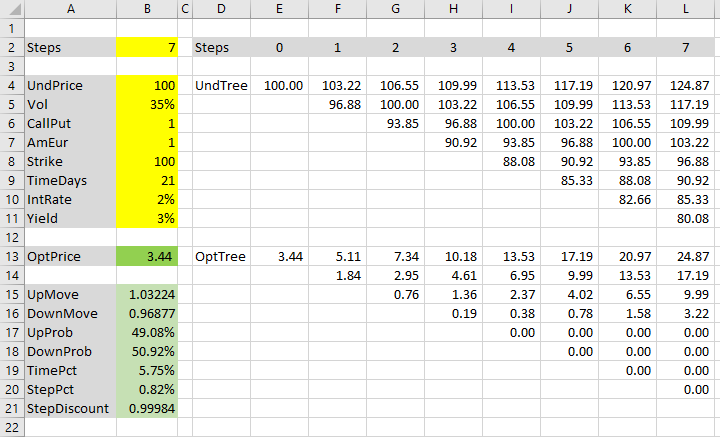Therefore we can use the same spreadsheet for multiple models. We just need to add some IFs to cells B15-B18, in order to use the correct formulas for the particular model.

Let's add a model selection input in cell B1 and define its name "Model".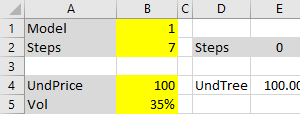This input will have the value 1 for Cox-Ross-Rubinstein (cells B15-B18 will use the existing CRR formulas) and 2 for Jarrow-Rudd (the formulas we will add now).

If you know how to create combo boxes, you can use one for the Model input cell.

## Up Move Probability

The main characteristic of the Jarrow-Rudd model (see all JR formulas and reference) is that up and down moves have the same probability, which of course must be 50%, because the two probabilities must add up to one. Jarrow-Rudd is sometimes called the equal probability model.

We will extend the up move probability formula in cell B17 as follows.

We will put the existing formula (Cox-Ross-Rubinstein up move probability, which we have created in the previous part):

``(EXP((IntRate-Yield)*StepPct)-DownMove)/(UpMove-DownMove)``

... inside an IF function with the condition Model=1:

``=IF(Model=1,(EXP((IntRate-Yield)*StepPct)-DownMove)/(UpMove-DownMove),0.5)``

If the condition is true (Model is Cox-Ross-Rubinstein), up move probability is calculated using the Cox-Ross-Rubinstein formula. If it is false (Model is Jarrow-Rudd), the probability is 0.5.

Test it by changing the values in cell B1.

If you see the #NAME! error, make sure you have defined all the cell names.

## Down Move Probability

We don't need to change the down move probability formula in cell B18, because it is:

``=1-UpProb``

... regardless of the model.

The probabilities are done. Let's move on to move sizes.

## Up Move Size

Like we just did with probability, we will extend our existing Cox-Ross-Rubinstein up move formula:

``EXP(Vol*SQRT(StepPct))``

... for two models, by placing it inside an IF function with Model=1 as condition. The formula in cell B15 will be:

``=IF(Model=1,EXP(Vol*SQRT(StepPct)),"JR")``

Let's replace "JR" with Jarrow-Rudd up move size. The formula is: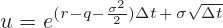... where:

• r is the risk-free interest rate – our IntRate input (cell B10)
• q is the dividend yield – our Yield input (cell B11)
• σ is volatility – our Vol input (cell B5)
• Δt is duration of one step – our StepPct cell (B20)

We already have all the inputs in our spreadsheet, so we can put them together. The formula is just a long yet simple expression inside an EXP function:

``=EXP((IntRate-Yield-Vol^2/2)*StepPct+Vol*SQRT(StepPct))``

So our final formula in cell B15 is:

``=IF(Model=1,EXP(Vol*SQRT(StepPct)),EXP((IntRate-Yield-Vol^2/2)*StepPct+Vol*SQRT(StepPct)))``

Alternatively, because both models calculate up move size as EXP of something, you can put the IF inside a single EXP. But I prefer the way shown above, which is clearer and allows us to add models with different formulas later.

## Down Move Size

In the same way, we will extend our existing down move formula in cell B16.

The Jarrow-Rudd down move formula is: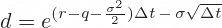The only difference is the minus sign before σ√Δt.

The Excel formula in cell B16 becomes:

``=IF(Model=1,1/UpMove,EXP((IntRate-Yield-Vol^2/2)*StepPct-Vol*SQRT(StepPct)))``

... where 1/UpMove is the Cox-Ross-Rubinstein down move formula calculated in the previous part.

If you are following the tutorial with the exact same inputs, the up and down move sizes with Model=2 should be 1.03164 and 0.96820, respectively.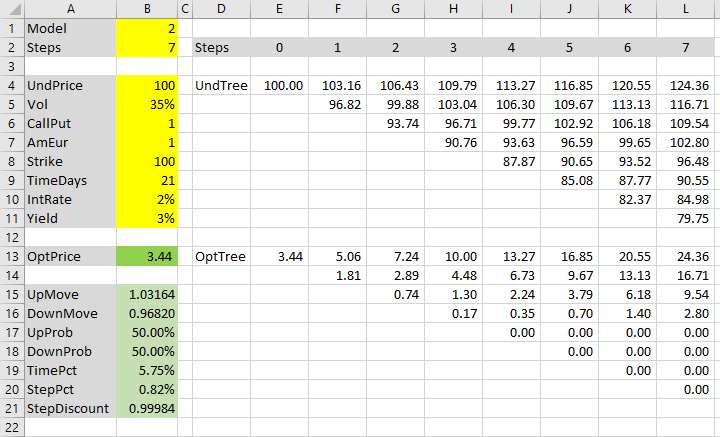## Binomial Trees and Option Price

If you have followed the tutorial from the beginning, you should have a working underlying price tree in cells E4-L11 and option price tree in cells E13-L20 (if not, see how to create them).

The resulting option price is in cell B13, which links to the first node in the option price tree (cell E13).

Now you can use the spreadsheet with either Cox-Ross-Rubinstein or Jarrow-Rudd model, switching between them in cell B1.

With our particular inputs, Jarrow-Rudd option price happens to be the same as Cox-Ross-Rubinstein option price (\$3.44), although some of the values in the binomial trees are slightly different. The two models are usually quite close, and their precision improves with growing number of steps.

## Next: Leisen-Reimer Model

The third and last model we will add to our spreadsheet is Leisen-Reimer. Its calculations are just a bit more complicated, but its advantage is that it tends to become more precise faster (with smaller number of steps), compared to either Cox-Ross-Rubinstein and Jarrow-Rudd.

Continue to Leisen-Reimer Model in Excel

By remaining on this website or using its content, you confirm that you have read and agree with the Terms of Use Agreement.

We are not liable for any damages resulting from using this website. Any information may be inaccurate or incomplete. See full Limitation of Liability.

Content may include affiliate links, which means we may earn commission if you buy on the linked website. See full Affiliate and Referral Disclosure.

We use cookies and similar technology to improve user experience and analyze traffic. See full Cookie Policy.